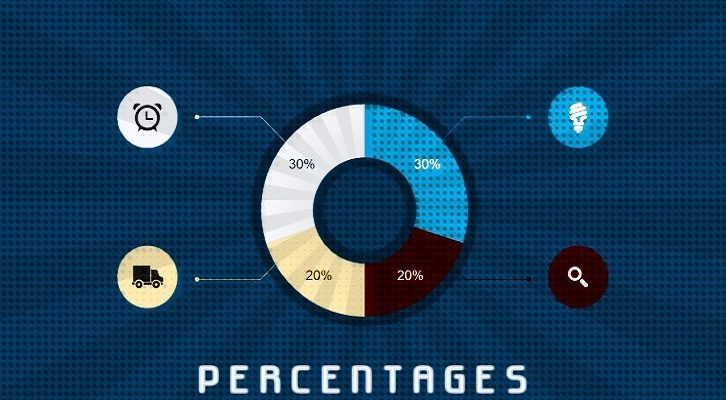# Percentages – Solutions

Have given below solutions to the first three questions on Percentages

1. a is x % of b, b is x% more than a. Find x?

a = bx,
b = a (1+x), substituting this in the previous equation, we get
x(x+1) = 1
x^2 + x -1 = 0

or x is approximately 0.62 or 62%

2. A two digit number ab is 60% of x. The two-digit number formed by reversing the digits of ab is 60% more than x. Find x?10a + b = 0.6x
10b + a = 1.6xSubtracting one from the other, we have9b – 9a = x or x = 9( b-a)
x should be a multiple of 9.10a + b = 3x/5, this implies that x should also be a multiple of 5.Or, x should be a multiple of 45

x should be equal to 45, ab = 27, ba = 72

3. A is x % more than B. B is y% less than C. If A,B and C are positive and A is greater than C, find the relation between x and y

a = (1 +x)b
b = c(1-y)
c = b /(1-y)

a is greater than c

Or, b (1+x) > b/(1-y)
1 + x > 1/(1-y)
(1+x)(1-y) > 1
1 + x -y – xy > 1
x > y (1+x)
y < x/(1+x)